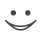# This flashcard is just one of a free flashcard set. See all flashcards!

130
Which of the following is true?
A.The futures rates calculated from a Eurodollar futures quote are always less than the corresponding forward rate
B.The futures rates calculated from a Eurodollar futures quote are always greater than the corresponding forward rate
C.The futures rates calculated from a Eurodollar futures quote should equal the corresponding forward rate
D.The futures rates calculated from a Eurodollar futures quote are sometimes greater than and sometimes less than the corresponding forward rate